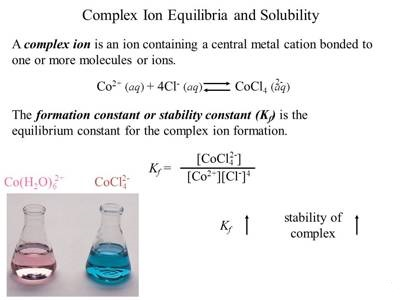Chemistry

# Equilibria of Complex IonsEquilibria of Complex Ions

Most transition elements and some non-transition metals are able to combine with neutral molecules or ions to form complex species. In many cases, complex ions are formed. Examples are Ag(NH3)2+, Cu(NH3)42+, HgI4, Zn(NH3)42+. The formation of complexes increases the solubility of certain sparingly soluble salts. For example, AgCI dissolves in excess ammonia solution, Cu(OH)2 is easily soluble in excess ammonia solution, mercuric iodide dissolves in excess potassium iodide solution. ZN(OH)2 dissolves in excess ammonia. As the metal ions form part of an anion or cation they lose their individual property and cannot usually be detected by qualitative tests, particularly if the complex ion is a stable one.In a solution containing the complex ion there is the equilibrium between the complex ion and the simple ions or molecules. Thus,

Ag(NH3)2+ + (aq) = Ag+ (aq) + 2NH3 (aq) and the equilibrium constant, using concentration is-

K1nst = [Ag+][NH3]2 / [Ag(NH3)2+]

This equilibrium represents the dissociation of the complex and the constant is called the instability constant. The reciprocal of Kinst i.e., the equilibrium constant of the reaction

Ag+ + 2 NH3 ↔ Ag (NH3)2+

is called the stability constant or formation constant. The stability constant gives a measure of the stability of the complex, since the higher its value, the greater the degree of formation, and hence the greater the stability of the complex ion.